Zener breakdown in a semi-conductor diode occurs when

(a) Forward current exceeds certain value

(b) Reverse bias exceeds certain value

(c) Forward bias exceeds certain value

(d) Potential barrier is reduced to zero

Concept Questions :-

Applications of PN junction
High Yielding Test Series + Question Bank - NEET 2020

Difficulty Level:

When forward bias is applied to a P-N junction, then what happens to the potential barrier ${V}_{\mathbit{B}}$, and the width of charge depleted region x

(a) ${V}_{\mathbit{B}}$ increases, x decreases

(b) ${V}_{\mathbit{B}}$ decreases, x increases

(c) ${V}_{\mathbit{B}}$ increases, x increases

(d) ${V}_{\mathbit{B}}$ decreases, x decreases

Concept Questions :-

PN junction
High Yielding Test Series + Question Bank - NEET 2020

Difficulty Level:

Function of rectifier is

(a) To convert ac into dc             (b) To convert dc into ac
(c) Both (a) and (b)                   (d) None of these

Concept Questions :-

Rectifier
High Yielding Test Series + Question Bank - NEET 2020

Difficulty Level:

When the P end of P-N junction is connected to the negative terminal of the battery and the N end to the positive terminal of the battery, then the P-N junction behaves like

(a) A conductor                       (b) An insulator
(c) A super-conductor              (d) A semi-conductor

Concept Questions :-

Applications of PN junction
High Yielding Test Series + Question Bank - NEET 2020

Difficulty Level:

A potential barrier of 0.50 V exists across a P-N junction. If the depletion region is $5.0×{10}^{-7}$m wide, the intensity of the electric field in this region is
(a)                       (b)
(c)                       (d)

Concept Questions :-

PN junction
High Yielding Test Series + Question Bank - NEET 2020

Difficulty Level:

If no external voltage is applied across P-N junction, there would be

(a) No electric field across the junction

(b) An electric field pointing from N-type to P-type side across the junction

(c) An electric field pointing from P-type to N-type side across the junction

(d) A temporary electric field during formation of P-N junction that would subsequently disappear

Concept Questions :-

PN junction
High Yielding Test Series + Question Bank - NEET 2020

Difficulty Level:

Barrier potential of a P-N junction diode does not depend on

(a) Temperature                (b) Forward bias
(c) Doping density             (d) Diode design

Concept Questions :-

PN junction

Difficulty Level:

Which is the correct diagram of a half-wave rectifier?

1.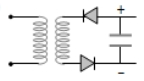2.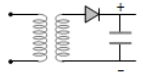3.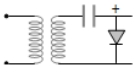4.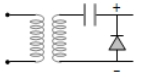Concept Questions :-

Rectifier

Difficulty Level:

The diode shown in the circuit is a silicon diode. The potential difference between the points A and B will be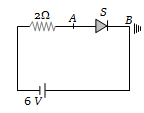(a) 6 V

(b) 0.6 V

(c) 0.7 V

(d) 0 V

Concept Questions :-

PN junction
High Yielding Test Series + Question Bank - NEET 2020

Difficulty Level:

Zener breakdown takes place if

(a) Doped impurity is low             (b) Doped impurity is high
(c) Less impurity in N-part           (d) Less impurity in P-type

Concept Questions :-

Applications of PN junction